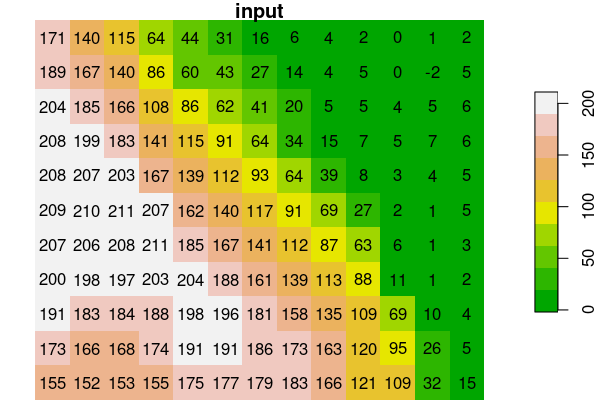Package starsExtra

R package starsExtra provides several miscellaneous functions for working with stars objects, mainly single-band rasters. Currently includes functions for:

• Focal filtering
• Detrending of Digital Elevation Models
• Calculating flow length
• Calculating the Convergence Index
• Calculating topographic slope
• Calculating topographic aspect

Installation

CRAN version:

install.packages("starsExtra")

GitHub version:

install.packages("remotes")
remotes::install_github("michaeldorman/starsExtra")

Usage

Once installed, the library can be loaded as follows:

library(starsExtra)
#> Linking to GEOS 3.8.0, GDAL 3.0.4, PROJ 6.3.1
#> Registered S3 methods overwritten by 'stars':
#>   method             from
#>   st_bbox.SpatRaster sf
#>   st_crs.SpatRaster  sf

Examples

Focal filter: aggregation functions

data(dem)
dem_mean3 = focal2(dem, matrix(1, 3, 3), "mean", na.rm = TRUE)
dem_sum3 = focal2(dem, matrix(1, 3, 3), "sum", na.rm = TRUE)
dem_min3 = focal2(dem, matrix(1, 3, 3), "min", na.rm = TRUE)
dem_max3 = focal2(dem, matrix(1, 3, 3), "max", na.rm = TRUE)
plot(dem, main = "input", text_values = TRUE, breaks = "equal", col = terrain.colors(10))
plot(dem, col = rep(NA, 3), key.pos = NULL, main = "")
plot(round(dem_mean3, 1), main = "mean (k=3)", text_values = TRUE, breaks = "equal", col = terrain.colors(10))
plot(dem_sum3, main = "sum (k=3)", text_values = TRUE, breaks = "equal", col = terrain.colors(10))
plot(dem_min3, main = "min (k=3)", text_values = TRUE, breaks = "equal", col = terrain.colors(10))
plot(dem_max3, main = "max (k=3)", text_values = TRUE, breaks = "equal", col = terrain.colors(10))